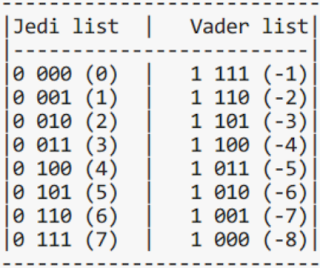## July 22, 2012

### 2's complement - The way computer counts.

Let's start first post with counting. Computers understand binary language which consists of only two symbols, basically represented as '0' and '1'. I assume you know how to represent decimal numbers into binary domain. I'm giving here a fictional story to make you understand how computer counts.
In binary world there are two persons, Jedi and Vader. Jedi stands for good and Vader is of evil. Jedi rules over '+'(positive) domain and Vader rules over '-'(negative) domain. Now both have to count but they are opposite to each other so they start with exact opposite ends(as they don't like each other). Jedi start with all 'zero' bits while counting in his positive domain and Vader start with all 'one' bits his domain. They have something in common(both are powerful) and that's why the pattern in which they counts is same but they use just opposite symbols. What is '0' to Jedi is '1' to Vader and vice versa. Below two lists are given. The numbers in list are of 4-bits(1 bit for sign, 3 bits for value) for demo. Entries in list are written as "s vvv". Here 's' is sign bit and 'v' is the data bit. Space between sign bit and data bits is just for sake of clarity. It has no other meaning. Left list is for Jedi and right list is for Vader. While counting, sign bit is always '0' for Jedi and '1' for Vader. As you can see the bit pattern is exactly same for these lists, except that they are opposite to each other in bit.  Number in parentheses is the corresponding value of that bit pattern.Suppose we've to find out how '5' is stored in computer. From Jedi list you'll find that it is "0101" but what about negative integers, say -4? Advantage of using 2's complement is we don't have to consider the Vader list. For -4 we'll look up the Jedi list which says "0100". As -4 in negative domain, so we've to flip all the bits which makes it "1011". Flipping the bits gives the corresponding number in negative(vader) domain but values in Vader list are alway '1' less than the Jedi list values. To compensate, we'll add '1' to the obtained bit pattern which is "1011" in this case. After adding it will become "1100". This is the 2's complement representation of -4.

This is fiction story but the computer uses the similar patterns as for values as given in list. Computer uses 'byte' which consists of 8 bits. With a byte(8-bit) you can count from 0 to +127 and -1 to -128 in the same pattern as of the above lists, total 256(1+127+128) different values. This whole setup is called 2's complement method which makes computer enable to store negative integers as well as positive integers.

Advantage of this notation is that computer don't have to use subtraction. Subtraction could be done using addition only. e.g. 3 - 7 = 3 + (-7) = -4. In binary (from the above lists), it would be 0011(3) + 1001(-7) = 1100(-4)

Happy learning :)